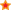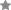for Secondary Education in the UK.Find Resources By Subjects
Information

# Numeracy for the Less Able

Price: £25.00
Age Range: KS3

Develop basic numeracy skills in students aiming for level 4. Worksheets focus on the requirements of the Year 7 Numeracy Framework and follow the three-part lesson idea introduced by the National Numeracy Strategy.

Processes include: Addition, subtraction, multiplication and division facts; Written and mental arithmetic methods; Solving problems; Doubling and halving; Rounding; Number patterns; Using a calculator.

Quantity Format
Size: 73 Pages
ISBN: 978 1 86025 401 7
Author(s): Graham Tipping
Code: NFTL
Popularity rank: 72
Average Customer Review:based on 3 reviews

# Contents List

#### LESSONS

• 9 SUBTRACTION Students will learn subtraction facts up to 10.
• 11 ADD OR SUBTRACT? Students will learn to use the appropriate operation when adding or subtracting.
• 13 IN THE RIGHT PLACE Students will develop use of the number facts to 10 using place value (units, tens and hundreds).
• 15 ADDITION 2 Students will learn addition facts up to 20.
• 17 SUBTRACTION 2 Students will learn subtraction facts up to 20.
• 19 UP TO 100 Students will gain practice with the complements of whole numbers up to 100.
• 21 DOUBLES AND HALVES Students gain practice in methods of doubling and halving numbers up to 100.
• 23 MULTIPLICATION Students will learn the 2, 3, 4, 5, and 10 times tables.
• 25 DIVISION Students will learn division facts associated with the 2, 3, 4, 5 and 10 times tables.
• 27 MULTIPLICATION AND DIVISION Students will be able to solve simple problems using multiplication and division facts of the 2, 3, 4, 5 and 10 times tables.
• 29 TEN AND HUNDRED Students will be able to multiply and divide by 10 and 100.
• 31 MULTIPLICATION 2 Students will learn multiplication facts up to 10 x 10.
• 33 DIVISION 2 Students will learn division facts up to 10 x 10.
• 35 SOLVING PROBLEMS Students will be able to use all four operations to solve simple problems.
• 37 DIVISION 3 Students will be able to test the divisibility of 2, 3, 5, 6, and 9.
• 39 WRITTEN ADDITION Students will be able to use standard written methods to add numbers up to three digits.
• 41 WRITTEN SUBTRACTION Students will be able to use standard written methods to subtract numbers to three digits (not including 'borrowing').
• 43 WRITTEN SUBTRACTION 2 Students will be able to use written methods for subtraction including 'borrowing' (decomposition).
• 45 ADDITION AND SUBTRACTION Students will be able to use addition as the inverse of subtraction to check answers to calculations.
• 47 WRITTEN MULTIPLICATION Students will be able to use standard written methods to multiply by a single digit.
• 49 WRITTEN DIVISION Students will be able to use standard written methods to divide by a single digit.
• 51 DIVISION WITH REMAINDERS Students will be able to use standard written methods to divide by a single digit, with remainders.
• 53 MULTIPLICATION OR DIVISION Students will be able to decide which to use - multiplication or division - to solve problems.
• 55 CHECKING ANSWERS Students will be able to use rounding to one significant figure, to check the reasonableness of results.
• 57 ODD AND EVEN Students will understand the number patterns obtained when adding and subtracting odd and even numbers.
• 59 USING A CALCULATOR EFFICIENTLY Students will be able to use a calculator to solve problems, including the use of brackets and memory.
• 61 USING A CALCULATOR EFFICIENTLY 2 Students will be able to interpret the calculator display within the context of a problem.
• 62 APPENDIX 1 for use with ADD OR SUBTRACT? page 11
• 63 APPENDIX 2 for use with SUBTRACTION 2, page 17 and DIVISION 2, page 33
• 64 APPENDIX 3 for use with MULTIPLICATION 2, page 31
• 66 APPENDIX 4 for use with DIVISION 2, page 33
• 68 APPENDIX 5 for use with MULTIPLICATION AND DIVISION, page 53
• 69 APPENDIX 6 for use with USING A CALCULATOR EFFICIENTLY, page 59
• 71 APPENDIX 7 Blank Speed Test grids

## Reviews

08/12/21

### at n/a

Excellent resource. Thank you.